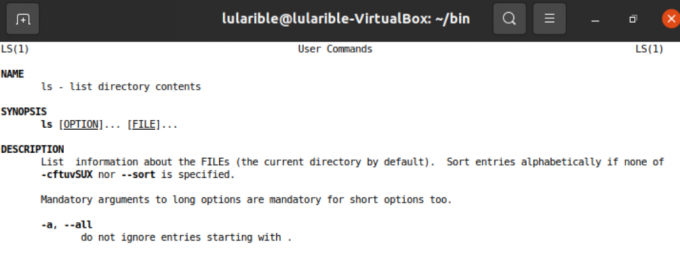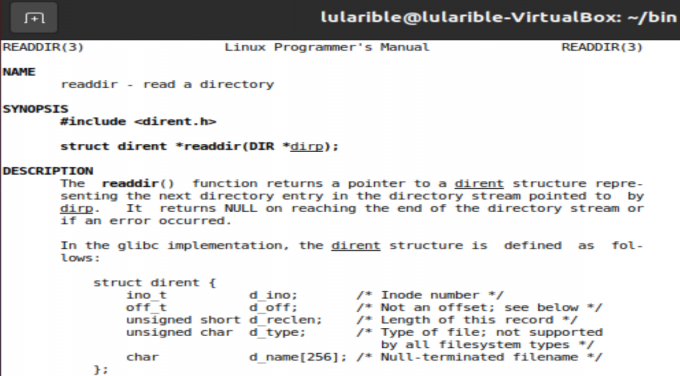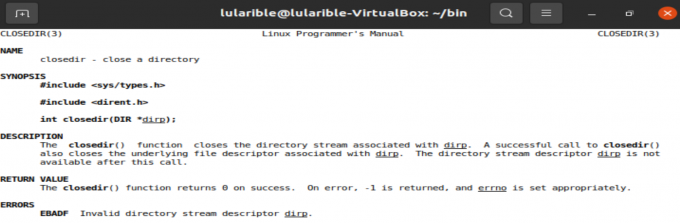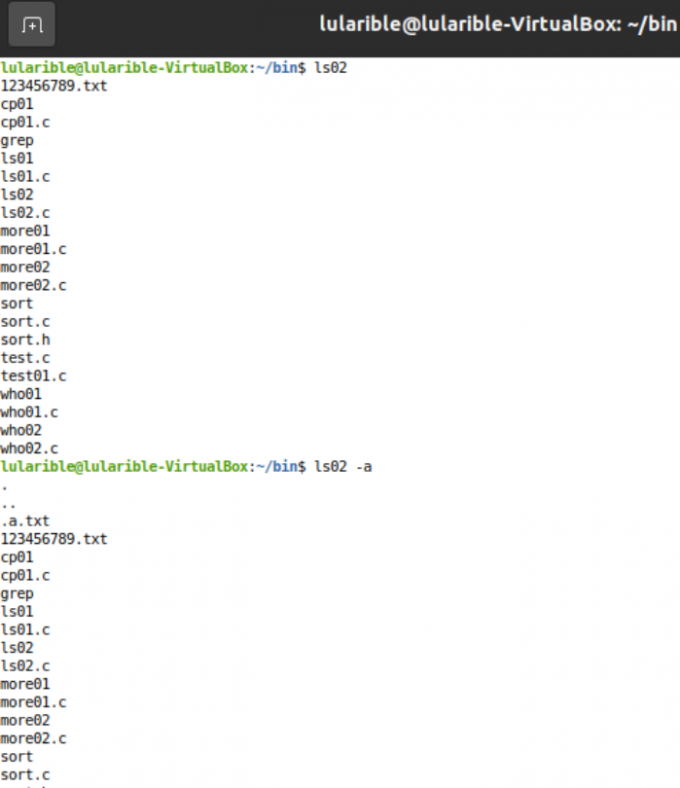# Linux系统编程【3.1】——编写ls命令

## ls命令简介

（有关于man命令的简介可以参考笔者前期博客：linux系统编程【1】——编写more命令)## 如何实现初级版ls命令

### 确定工具函数### 确定所需参数

1.opendir打开指定目录

3.打印结构体中的d_name字符串

}

4.closedir关闭指定目录

### ls命令源代码

``````/*
* ls01.c
* writed by lularible
* 2021/02/07
*/
#include<stdio.h>
#include<stdlib.h>
#include<sys/types.h>
#include<dirent.h>
#include<string.h>

//函数声明
void do_ls(const char*);
void show_ls(struct dirent*);
void error_handle(const char*);

int main(int argc,char* argv[])
{
//对输入的命令进行参数判断与处理
if(argc == 1){	//只输入了：ls
do_ls(".");
}
else{
while(--argc){
do_ls(*(++argv));
}
}
return 0;
}

//主流程
void do_ls(const char* dir_name)
{
DIR* cur_dir;
struct dirent* cur_item;
//打开目录
if((cur_dir = opendir(dir_name)) == NULL){
error_handle(dir_name);
}
else{
//读取目录并显示信息
show_ls(cur_item);
}
printf("\n");
//关闭目录
closedir(cur_dir);
}
}

//显示文件名
void show_ls(struct dirent* cur_item){
printf("%s",cur_item->d_name);
printf("\n");
}

//错误处理
void error_handle(const char* dir_name){
perror(dir_name);
exit(1);
}
``````

## 增加可选参数"-a"和排序

• 1.当不带任何参数时，显示的文件名不包括隐藏文件（以'.'开头的文件名），带上参数"-a"时，才把隐藏的文件名显示出来

• 2.将文件名先按照字典序排序再显示

### 源代码

``````/*
* ls02.c
* writed by lularible
* 2021/02/07
*/

#include<stdio.h>
#include<stdlib.h>
#include<sys/types.h>
#include<dirent.h>
#include<string.h>
#include</home/lularible/bin/sort.h>	//字典序排序算法

//函数声明
void do_ls(const char*);
void restored_ls(struct dirent*);
void error_handle(const char*);

//全局变量
int has_a = 0;				//标记是否带'-a'参数
char *filenames;		        //存放文件名
int file_cnt = 0;			//目录中文件个数

int main(int argc,char* argv[])
{
//对输入的命令进行参数判断与处理
if(argc == 1){	//只输入了：ls
do_ls(".");
}
else{
if(strcmp(argv,"-a") == 0){
has_a = 1;
--argc;
++argv;
}
if(argc == 1){
do_ls(".");
}
else{
while(--argc){
do_ls(*(++argv));
}
}
}
return 0;
}

void do_ls(const char* dir_name)
{
DIR* cur_dir;
struct dirent* cur_item;
//打开目录
if((cur_dir = opendir(dir_name)) == NULL){
error_handle(dir_name);
}
else{
//读取目录并显示信息
//将文件名存入数组
restored_ls(cur_item);
}
//字典序排序
sort(filenames,0,file_cnt-1);
//输出结果
int i = 0;
for(i = 0;i < file_cnt;++i){
printf("%s\n",filenames[i]);
}
//关闭目录
closedir(cur_dir);
}
}

void restored_ls(struct dirent* cur_item){
char* result = cur_item->d_name;
//当不带-a参数时，隐藏以'.'开头的文件
if(!has_a && *result == '.')	return;
filenames[file_cnt++] = cur_item->d_name;
}

void error_handle(const char* dir_name){
perror(dir_name);
exit(1);
}
``````

``````/*
* sort.h
* writed by lularible
* 2021/02/07
*/

#include<stdio.h>
#include<stdlib.h>
#include<string.h>

void swap(char** s1,char** s2);
int compare(char* s1,char* s2);
int partition(char** filenames,int start,int end);
void sort(char** filenames,int start,int end);

//交换两字符串
void swap(char** s1,char** s2)
{
char* tmp = *s1;
*s1 = *s2;
*s2 = tmp;
}

//比较两字符串的字典序
//s1靠前，返回负数，s1靠后，返回正数
//s1和s2完全一样，返回0
int compare(char* s1,char* s2){
while(*s1 && *s2 && *s1 == *s2){
++s1;
++s2;
}
return *s1 - *s2;
}

int partition(char** filenames,int start,int end){
if(!filenames)	return -1;
char* privot = filenames[start];
while(start < end){
while(start < end && compare(privot,filenames[end]) < 0)
--end;
swap(&filenames[start],&filenames[end]);
while(start < end && compare(privot,filenames[start]) >= 0)
++start;
swap(&filenames[start],&filenames[end]);
}
return start;
}

void sort(char** filenames,int start,int end){
if(start < end){
int position = partition(filenames,start,end);
sort(filenames,start,position - 1);
sort(filenames,position + 1,end);
}
}
``````

## 效果展示## 参考资料

《Understanding Unix/Linux Programming A Guide to Theory and Practice》

### 精彩评论换一张